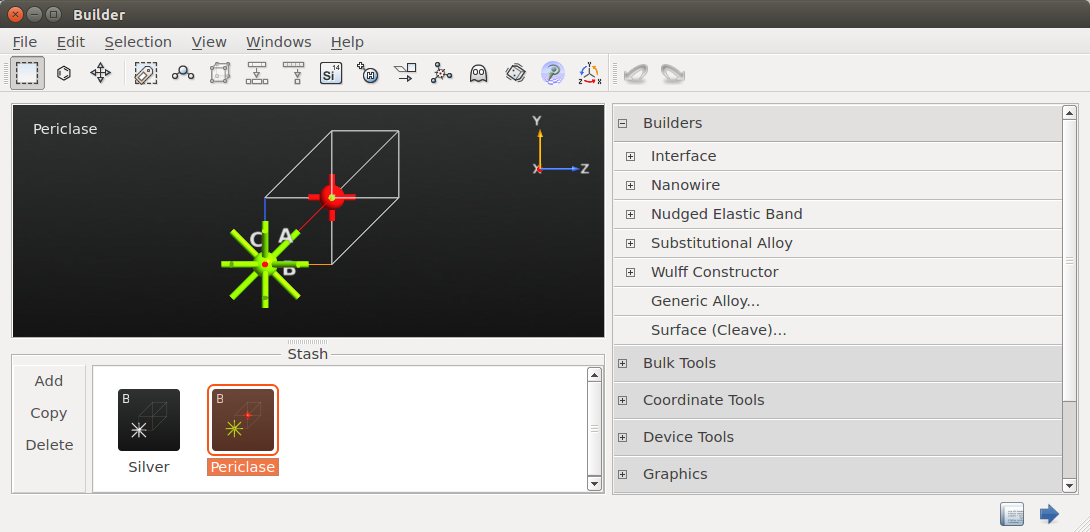# The Interface Builder in QuantumATK¶

PDF version

The Interface Builder of QuantumATK allows the construction of complex interfaces by automatically matching the 2D unit cells of two different surfaces and compare the strain. The input is two crystal surfaces which can be created from bulk systems using the Surface (Cleave) tool. A specified number of atomic layers can be added for each material, an atomic layer being defined as the atoms that lie with the same distance to the AB-plane of the unit cell.Fig. 185 The interface builder used on InAs(111) and Al(111).

## Select Surface Cells¶

The Select Surface Cells button is used to view the results of the lattice matching. This opens a window where the strain of all the found matches is plotted against the total number of atoms in the interface unit cell, see Fig. 186. The selected unit cell is the red dot, one can select alternative matches by clicking the blue dots.Fig. 186 The Select Surface Cells window.Fig. 187 The matching of two surface lattices. $$(a_1,a_2)$$ and $$(b_1,b_2)$$ are the unit cell vectors of the two surfaces, and $$(c_1,c_2)$$ is the rotated cell of the b surface. $$v_1$$ and $$v_2$$ are a linear combination of $$c_1$$ and $$c_2$$. $$u_1$$ and $$u_2$$ are $$v_1$$ and $$v_2$$, respectively, truncated to the grid of cell a.

The procedure for matching the two lattices is as follows;

• The unit cell vectors of surface a $$(a_1,a_2)$$ and b $$(b_1,b_2)$$ are extracted.

• The b cell is rotated by an angle $$\theta$$, $$(c_1,c_2)$$ in Fig. 187. This is done for a set of angles $$\theta \in [\theta_{min};\theta_{max}]$$ with an default increment of $$\delta_\theta = 4$$ degrees. For each of the rotations;

• All possible lattice vectors, $$v$$, where $$(c_1,c_2)$$ has been repeated a maximum of $$(n^{max},m^{max})$$ times is found, $$v_1$$ or $$v_2$$ in Fig. 187.

$v = i\cdot c_1+j \cdot c_2, \qquad i \in [-n^{max};n^{max}], \, j \in [0,m^{max}].$
• We express $$v$$ in the lattice of cell a by creating a linear transformation,

$\begin{split}U = \begin{pmatrix} a_{1,x} & a_{2,x} \\ a_{1,y} & a_{2,y} \end{pmatrix}, \qquad v = U s,\end{split}$

where $$s = U^{-1}v$$ describes how $$v$$ is expressed in the unit cell vectors $$a_1$$ and $$a_2$$.

• The vector, $$v$$, needs to be truncated to the grid of a. This is done by rounding the elements of $$s$$ to integers, $$s'$$, and calculating

\begin{split}\begin{aligned} u &= U s'.\end{aligned}\end{split}

$$u$$ ($$u_1$$ or $$u_2$$ in Fig. 187) corresponds to the lattice vector of cell a that $$v$$ will be matched to.

• All possible cells, $$(v_1,v_2)$$, of b is created by iterating through all the found lattice vectors $$v$$ two by two.

• The strain tensor from the $$(v_1,v_2)$$ cell to the corresponding $$(u_1,u_2)$$ cell is calculated. This can be done using the following three straining methods; only straining $$(v_1,v_2)$$, only straining $$(u_1,u_2)$$, or straining both equally. The method can by chosen in the Select Surface Cells window. For the first straining method, we get

\begin{split}\begin{aligned} \varepsilon_{11} &= \left| \frac{v_{1,x}}{u_{1,x}} \right| - 1. \\ \varepsilon_{22} &= \left| \frac{v_{2,y}}{u_{2,y}} \right| - 1. \\ \varepsilon_{12} &= \frac{1}{2} \frac{v_{2,x}-\frac{v_{1,x}}{u_{1,x}}u_{2,x}}{u_{2,y}}.\end{aligned}\end{split}

Equivalent formulas are used for the second straining method. If both surfaces should be strained equally, an intermediate cell is created between the $$(v_1,v_2)$$ and $$(u_1,u_2)$$ cell. This cell in constructed s.t. the strain tensor from the first lattice to the intermediate one is exactly minus the stress tensor from the second to the intermediate one. From the stress tensor, we find the mean absolute stress $$\varepsilon^{av}$$,

\begin{aligned} \varepsilon^{av} = \frac{\varepsilon_{11}+\varepsilon_{22}+\varepsilon_{12}}{3}.\end{aligned}

Lattices with a mean absolute stress outside the specified limits $$\varepsilon^{av} \in [\varepsilon_{min}; \varepsilon_{max}]$$ are discarded and strains within a specified $$tolerance$$ are considered equal.

• The total number of atoms in the corresponding interface is found by considering the area of the cell and the lattice match is ranked according to the following score, $$S$$;

\begin{split}\begin{aligned} S_{\phi} &= \sum_{\alpha} 1-7\mathrm{e}^{-\frac{(\phi-\alpha)^2}{15}}, \\ \alpha &\in [15,30,45,60,90,120,150] \, \mathrm{degrees}, \\ S &= \mathrm{e}^{-\varepsilon^{av}-\frac{A}{10}-S_{\phi}},\end{aligned}\end{split}

where $$\phi$$ is the angle between the two vectors of the cell, and $$A$$ is the area of the cell. This score favours the cells where the angle between the vectors are close to any of the angles in $$\alpha$$, the mean absolute strain is low and the area is small. This is based on the assumption that a small unit cell will be energetically favourable and that a low strain will minimize the amount of defects. The score is a subjective number, created to have a default guess for the interface, and is therefore not meant as a general way of predicting the most physically sensible interface.

• The lattice match with the best score will be the default choice of the builder.

The matching parameters can be changed using the Set Matching Parameters button in the Select Surface Cells window, see Fig. 188. The default parameters are listed in the table below.

 $$n^{max}$$ 6 $$m^{max}$$ 6 $$\theta_{min}$$ 0 degrees $$\theta_{max}$$ 180 degrees $$\delta_{\theta}$$ 4 degrees $$\varepsilon_{min}$$ -100 % $$\varepsilon_{max}$$ 100 % $$tolerance$$ $$1^{-6}$$Fig. 188 The Set Matching Parameters window.

## Shift Surfaces¶

When a suitable lattice match has been found, the Shift Surfaces button can be used to shift one surface w.r.t. to the other. This can be done either manually or by minimizing the energy of the system. Using the second option, the geometry is optimized using a classical potential from the QuantumATK Classical package and with the constrains that each material acts as a rigid body. The optimization method is LBFGS and the optimization procedure ends when the inter-atomic forces are below 0.02 eV/Å. The calculated displacement vector is shown in the window together with the total energy of the system, if the Calculate energy checkbox is checked. The displacement is automatically applied to the interface. It should be noted, that his procedure only finds local minima of which there could be several. To find the global minima one should optimize the structure using several different starting points determined by the symmetry.